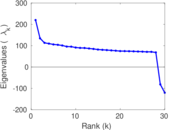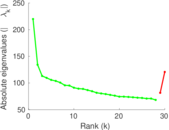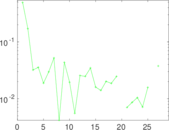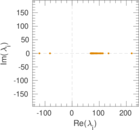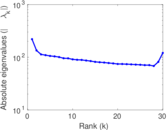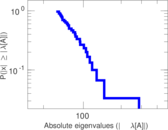This network consists of the wikilinks of the Wikipedia in the Spanish language (es). Nodes are Wikipedia articles, and directed edges are wikilinks, i.e., hyperlinks within one wiki. In the wiki source, these are indicated with [[double brackets]]. Only pages in the article namespace are included.

 Code `Wes` Internal name `wikipedia_link_es` Name Wikipedia links (es) Data source http://dumps.wikimedia.org/ AvailabilityDataset is available for download Consistency checkDataset passed all tests Category Hyperlink network Node meaning Article Edge meaning Wikilink Network format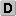Unipartite, directed Edge typeUnweighted, no multiple edges ReciprocalContains reciprocal edges Directed cyclesContains directed cycles Loops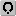Contains loops

## Statistics

 Size n = 3,033,373 Volume m = 46,656,045 Loop count l = 3,578 Wedge count s = 504,330,438,746 Claw count z = 21,791,298,823,525,412 Cross count x = 9.525 47 × 1020 Triangle count t = 187,642,529 Maximum degree dmax = 275,173 Maximum outdegree d+max = 4,332 Maximum indegree d−max = 275,153 Average degree d = 30.761 8 Fill p = 5.070 56 × 10−6 Size of LCC N = 3,032,808 Size of LSCC Ns = 1,724,003 Relative size of LSCC Nrs = 0.568 345 Diameter δ = 10 50-Percentile effective diameter δ0.5 = 3.252 29 90-Percentile effective diameter δ0.9 = 4.269 95 Median distance δM = 4 Mean distance δm = 3.722 75 Balanced inequality ratio P = 0.203 592 Outdegree balanced inequality ratio P+ = 0.228 732 Indegree balanced inequality ratio P− = 0.148 632 Tail power law exponent γt = 2.521 00 Degree assortativity ρ = −0.098 352 8 Degree assortativity p-value pρ = 0.000 00 Clustering coefficient c = 0.001 116 19 Directed clustering coefficient c± = 0.031 040 0 Spectral norm α = 1,349.38 Operator 2-norm ν = 1,330.45 Cyclic eigenvalue π = 219.828 Reciprocity y = 0.120 383 Non-bipartivity bA = 0.022 150 4 Normalized non-bipartivity bN = 0.008 366 30

## Plots

### Degree distribution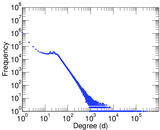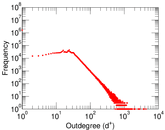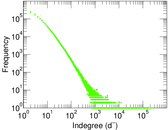### Cumulative degree distribution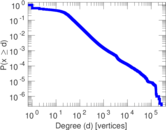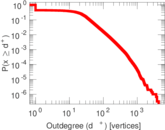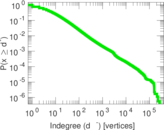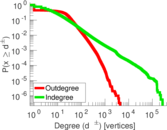### Lorenz curve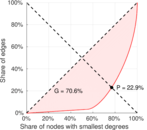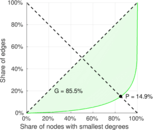### Zipf plot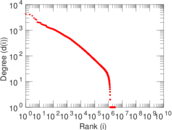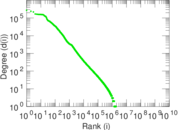### Hop distribution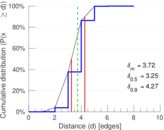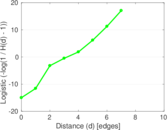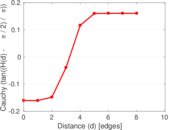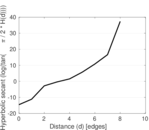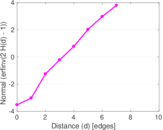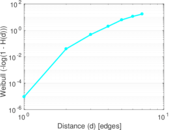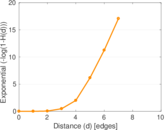### In/outdegree scatter plot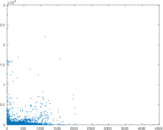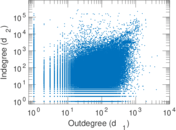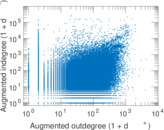### Matrix decompositions plots